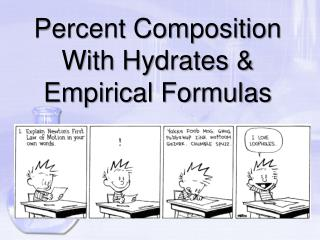DownloadDownload PresentationPercent Composition With Hydrates & Empirical Formulas

# Percent Composition With Hydrates & Empirical Formulas

Télécharger la présentation## Percent Composition With Hydrates & Empirical Formulas

- - - - - - - - - - - - - - - - - - - - - - - - - - - E N D - - - - - - - - - - - - - - - - - - - - - - - - - - -
##### Presentation Transcript

1. Percent Composition With Hydrates & Empirical Formulas

2. HW – Ques. 7.4 Calculate the percent composition to the nearest whole number of each of the following compounds. CO ZnSiO3 H3PO4 Ca(NO3)2

3. What is a Hydrate? • Water included in the crystal (crystallized compounds containing water) • Heating removes the water methane hydrate

4. Hydrates CuSO4 · 5H2O = copper (II) sulfate pentahydrate CuSO4 · 5H2O water loosely attached heat CuSO4 Anhydrate – no water (white) Hydrate (blue)

5. Finding % Water In Hydrates CuSO4 · 5H2O Step 1: Cu S O 160 H O 90 160 +90 250 Step 2: 1 X 64 = 64 1 X 32 = 32 Step 3: 4 X 16 = 64 Find % H2O 10 X 1 = 10 90 250 = 36% X 100% 5 X 16 = 80

6. Finding % Water In Hydrates Na2CO3· 10 H2O Step 1: Na C O 106 H O 180 106 +180 286 Step 2: 2 X 23 = 46 1 X 12 = 12 Step 3: 3 X 16 = 48 Find % H2O 20 X 1 = 20 180 286 = 63% X 100% 10 X 16 = 160

7. Empirical Formula • The lowest whole number ratio Empirical Formula HO CH4 CH2O CH H2O Molecular Formula H2O2 C2H8 C6H12O6 C6H6 H2O

8. Finding Empirical Formulas • Reverse of determining % composition • Divide the percent of each element by the atomic mass of the element (g/mol) • Assume 100g  % is changed to grams • If mole ratio (answers) can not be converted to whole numbers easily, divide each by the smallest number answer • If answer is still not in whole numbers, multiply the answers by 2, 3, 4, … until you get whole numbers

9. Find the Empirical Formula A compound of C, H, & O has the following composition by mass: C - 48.64%, H - 8.16%, O - 43.20% Step 1: 1 mol C 12.011 g C x = 4.050 mol C 48.64 g C 1 mol H 1.007 g H 8.16 g H = 8.103 mol H x 1 mol O 15.999 g O 43.20 g O x = 2.700 mol O

10. 2.700 2.700 8.103 2.700 Step 2: C?H?O? C H O C1.5H3O1 Step 3: Multiply by 2, 3, 4, … until whole # reached Answer: C3H6O2 4.050 2.700

11. Find the Empirical Formula C – 62.1%, H – 13.8%, N – 24.1% 1 mol C 12 g C 62.1 g C = 5.175 mol C x 1 mol H 1 g H 13.8 g H x = 13.8 mol H 1 mol N 14 g N 24.1 g N = 1.72 mol N x 5.175 1.72 13.8 1.72 1.72 1.72 C H N C3H8N